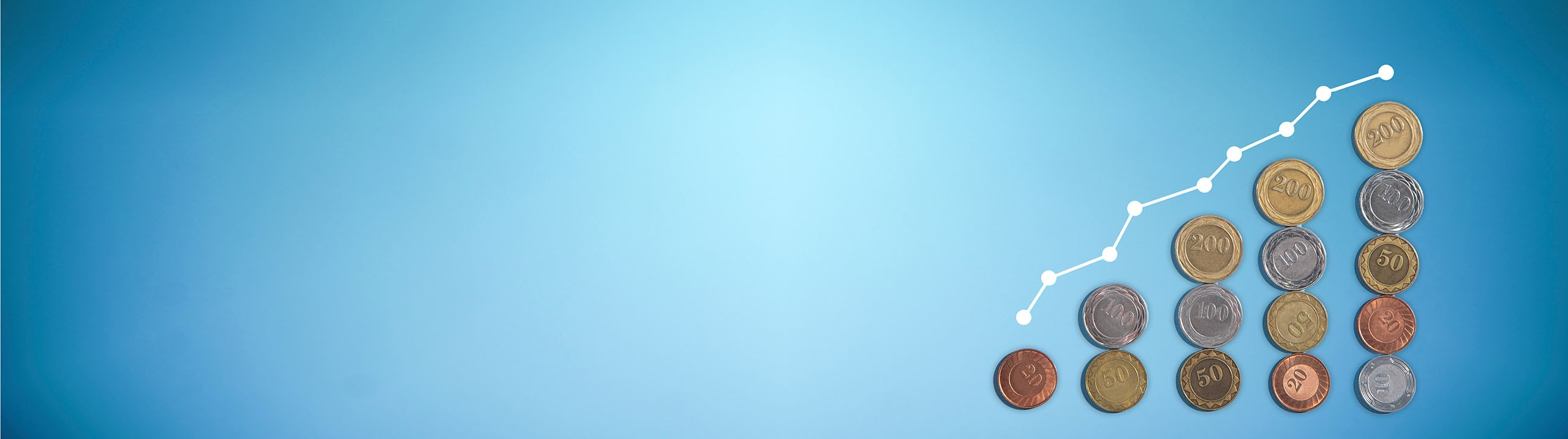Start typing, then use the up and down arrows to select an option from the list.# Macroeconomics

Learn the toughest concepts covered in your Macroeconomics class with step-by-step video tutorials and practice problems.

Unemployment and Inflation

# Demand-Pull and Cost-Push Inflation

1

#### concept

Demand-Pull Inflation3m
Play a video:
Alright now let's see how inflation relates to our supply and demand graph. So inflation can be caused by both demand or supply. So an increased demand or a decreased supply. So the first one, let's talk about demand, the demand side here, and we call it demand pull inflation because the demand is pulling the prices upward were on the supply side, the supply is gonna be supply push and the supply is pushing them upwards. Okay, so the demand pull inflation, the idea here is that there's too much spending, too much spending chasing too few goods, so there's a lot of demand but not enough supply to match it, right? So in this case the demand is pulling the price levels higher and if we're gonna hold production constant, let's say that we can't increase the supply right now, uh the supply is going to be constant, but the demand is more and more, we're gonna see the prices increase. Okay, so let's go to our our our graph here and let's see our standard market graph here, where we've got our downward demand And our upward supply here, right? We'll label them D one and S one and we're gonna say that demand increases here, right, there's so much demand in the market that the demand is increasing and we're gonna draw a new demand curve to the right, right? When demand is increasing, we would have a new demand curve to the right, okay, so what we would expect is for us to move to this new equilibrium where our original equilibrium was right here, we would expect a new equilibrium right there, Right? So at our old equilibrium we had Q. One right here and Q. Two. Excuse me, Price price one here and then the the new equilibrium would have a higher quantity and a higher price, right? So we would see an increase in prices because of this increased demand. But that's normal, we would expect that. But when inflation happens, well, guess what? We're gonna hold the supply constant, the supply can't increase. We can't have this increase in the quantity to match the increased demand. There's no there's no more available output in in our our society or whatever. So let's go ahead and see um how this affects it. So if we've got this new demand, so that that was the ideal, what would be happening. But in fact we've got this new demand over here where this would have been the equilibrium. However the quantity supplied can't go past this original point. There's no way to meet the new demand and we're gonna have the quantity supplied staying here at the original point. Let me get out of the way. So we can see the rest of the graph, the quantity supplied is at that point. Um So what do we see that the demand is going to be over here? Right. The demand is over here on our new graph, um willing to pay a higher price for that for that level of supply. Okay, so we would see this higher level of supply. Um Excuse me. This higher price level I'll put P. H. For the high price willing to pay at that quantity supply that's not able to increase. So notice what's happening. We had our equilibrium price down here P. Star and now we've got inflated prices. So you're not gonna probably have to draw this on the graph like this. I was just giving you more of a visual illustration. Um But more likely you're gonna have to just know what demand pull inflation is and that's the idea of increasing demand but the supply can't meet it. So the prices are gonna go up even though there's not more production. Okay so the demand is pulling the prices up and in the other case that we're going to see is that the cost is pushing the prices up. Okay so let's go ahead and talk about cost push inflation in the next
2

#### concept

Cost-Push Inflation2m
Play a video: